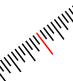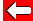# Area QuestionsGet a pencil and paper together - let's see what you know:
 How many square millimetres are there in a square centimetre? How many square centimetres are there in a square metre? How many square metres are there in a square centimetre?

When you've worked out the answers, go to the next page.Go back a pageMaths MenuGo to next page